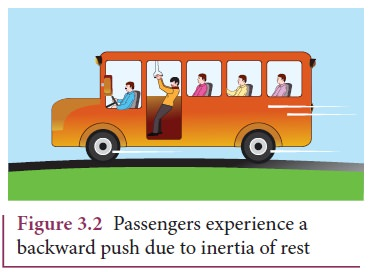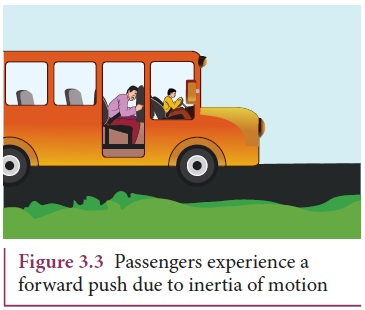Home | | Physics 11th std | Newton’s First Law

# Newton’s First Law

Every object continues to be in the state of rest or of uniform motion (constant velocity) unless there is external force acting on it.

Newton’s First Law

Every object continues to be in the state of rest or of uniform motion (constant velocity) unless there is external force acting on it.

This inability of objects to move on its own or change its state of motion is called inertia. Inertia means resistance to change its state. Depending on the circumstances, there can be three types of inertia.

1. Inertia of rest: When a stationary bus starts to move, the passengers experience a sudden backward push. Due to inertia, the body (of a passenger) will try tocontinue in the state of rest, while the bus moves forward. This appears as a backward push as shown in Figure 3.2. The inability of an object to change its state of rest is called inertia of rest.

2. Inertia of motion: When the bus is in motion, and if the brake is applied suddenly, passengers move forward and hit against the front seat. In this case, the bus comes to a stop, while the body (of a passenger) continues to move forward due to the property of inertia as shown in Figure 3.3. The inability of an object to change its state of uniform speed (constant speed) on its own is called inertia of motion.3. Inertia of direction: When a stone attached to a string is in whirling motion, and if the string is cut suddenly, the stone will not continue to move in circular motion but moves tangential to the circle as illustrated in Figure 3.4.This is because the body cannot change its direction of motion without any force acting on it. The inability of an object to change its direction of motion on its own is called inertia of direction.

When we say that an object is at rest or in motion with constant velocity, it has a meaning only if it is specified with respect to some reference frames. In physics, any motion has to be stated with respect to a reference frame. It is to be noted that Newton’s first law is valid only in certain special reference frames called inertial frames. In fact, Newton’s first law defines an inertial frame.

## Inertial Frames

If an object is free from all forces, then it moves with constant velocity or remains at rest when seen from inertial frames. Thus, there exists some special set of frames in which if an object experiences no force it moves with constant velocity or remains at rest. But how do we know whether an object is experiencing a force or not? All the objects in the Earth experience Earth’s gravitational force. In the ideal case, if an object is in deep space (very far away from any other object), then Newton’s first law will be certainly valid. Such deep space can be treated as an inertial frame. But practically it is not possible to reach such deep space and verify Newton’s first law.

For all practical purposes, we can treat Earth as an inertial frame because an object on the table in the laboratory appears to be at rest always. This object never picks up acceleration in the horizontal direction since no force acts on it in the horizontal direction. So the laboratory can be taken as an inertial frame for all physics experiments and calculations. For making these conclusions, we analyse only the horizontal motion of the object as there is no horizontal force that acts on it. We should not analyse the motion in vertical direction as the two forces (gravitational force in the downward direction and normal force in upward direction) that act on it makes the net force is zero in vertical direction. Newton’s first law deals with the motion of objects in the absence of any force and not the motion under zero net force. Suppose a train is moving with constant velocity with respect to an inertial frame, then an object at rest in the inertial frame (outside the train) appears to move with constant velocity with respect to the train (viewed from within the train). So the train can be treated as an inertial frame. All inertial frames are moving with constant velocity relative to each other. If an object appears to be at rest in one inertial frame, it may appear to move with constant velocity with respect to another inertial frame. For example, in Figure 3.5, the car is moving with uniform velocity v with respect to a person standing (at rest) on the ground. As the car is moving with constant velocity with respect to ground to the person is at rest on the ground, both frames (with respect to the car and to the ground) are inertial frames.Suppose an object remains at rest on a smooth table kept inside the train, and if the train suddenly accelerates (which we may not sense), the object appears to accelerate backwards even without any force acting on it. It is a clear violation of Newton’s first law as the object gets accelerated without being acted upon by a force. It implies that the train is not an inertial frame when it is accelerated. For example, Figure 3.6 shows that car 2 is a non-inertial frame since it moves with accelerationwith respect to the ground.These kinds of accelerated frames are called non-inertial frames. A rotating frame is also a non inertial frame since rotation requires acceleration. In this sense, Earth is not really an inertial frame since it has self-rotation and orbital motion. But these rotational effects of Earth can be ignored for the motion involved in our day-to-day life. For example, when an object is thrown, or the time period of a simple pendulum is measured in the physics laboratory, the Earth’s self-rotation has very negligible effect on it. In this sense, Earth can be treated as an inertial frame. But at the same time, to analyse the motion of satellites and wind patterns around the Earth, we cannot treat Earth as an inertial frame since its self-rotation has a strong influence on wind patterns and satellite motion.

Study Material, Lecturing Notes, Assignment, Reference, Wiki description explanation, brief detail
11th Physics : UNIT 3 : Laws of Motion : Newton’s First Law |